Figure 16.1 A 3D projection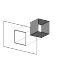R code
 Figure 16.2 A 3D viewpoint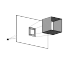R code
 Figure 16.3 A 3D surface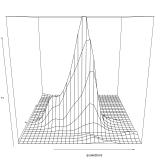R code
 Figure 16.4 An improved 3D surface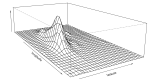R code
 Figure 16.5 A shaded 3D surface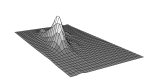R code
 Figure 16.6 A 3D scatterplot from lattice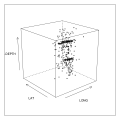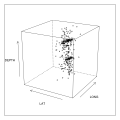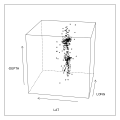R code
 Figure 16.7 A 3D scatterplot from scatterplot3d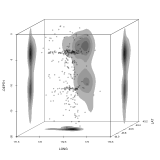R code
 Figure 16.8 A 3D surface plot from rgl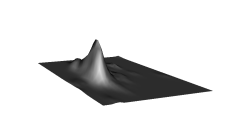R code
 Figure 16.9 A 3D contour plot of earthquakes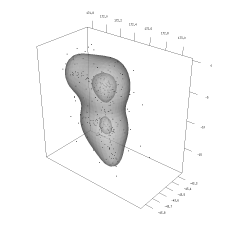R code
 Figure 16.10 A 3D image constructed from basic shapes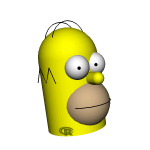R code
 Figure 16.11 A 3D image in VRML format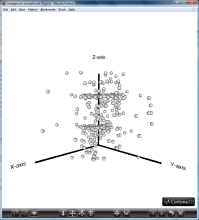R code